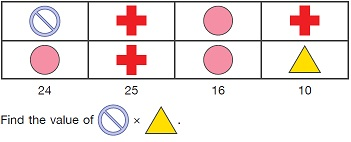# User ForumSubject :IMO    Class : Class 3

Study the diagram below. Each figure stands for a number.A6
B30
C12
D40

if you could explain the answer?

## Ans 1:

Class : Class 5
red circle= * =5*5 =25, o*o=4*4=16multiply each number in column. Answer will be 6*2=12

Class : Class 3
C

## Ans 3:

Class : Class 5
Don't just shout . See the answer.

## Ans 4:

Class : Class 6
I didn't understand . Someone explain. I want master answer or simple answer of Question 31 by Shiven .

## Ans 5:

Class : Class 6
How? Can you explain?

## Ans 6:

Class : Class 5
Oh! Now I know! answer is C as 6 * 2 = 12! (Key - * - Multiplication sign)

Class : Class 3

Class : Class 7